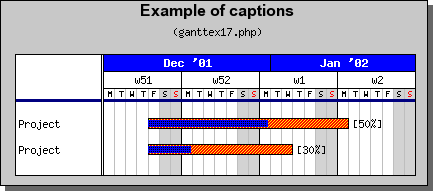Contents Previous Next

### 10.12.2 Modifying the divider lines

The vertical and horizontal lines between the titles and the bars can be modified by accessing the 'divider' and 'dividerh' properties of the scale. Again, this is straightforward as the following example shows:

```  \$graph->scale->divider ->SetWeight(3); \$graph->scale-> divider-> SetColor( "navy"); \$graph->scale-> dividerh-> SetWeight(3); \$graph->scale-> dividerh-> SetColor( "navy"); ```

The effect of this is shown in Figure 163 belowFigure 163: Modifying the dividing line [src]

Contents Previous Next